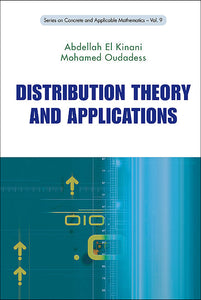# Distribution Theory And Applications

Abdellah El-kinani

Format: Print Book

ISBN: 9789814304917

• SGD 81.32
Unit price per
Tax included.

This book is an introductory course to the very important theory of distributions, as well as its applications in the resolution of partial differential equations (PDEs). It begins with a chapter of general interest, on the fundamental spaces (or test function spaces). The book advances and concludes with a chapter on Sobolev spaces, which are known to be very important in the resolution of PDEs.The very basic properties of distributions are examined in detail. Several formal methods have been first used, without rigorous justifications (Dirac function, principal value of Cauchy, finite parts of Hadamard). They find their natural frame in distribution theory. It is the same for Laplace transformation which is a fundamental tool in symbolic calculations.A detailed treatment is given to the convolution product for it is a central theme in distribution theory. Another very important instrument, covered in several chapters, is the Fourier transformation which is among the most fundamental tools in different mathematical disciplines, and also in physics. Convolution algebras, which are sufficient for the treatment of classical PDEs, are used in various applications.The general frame for the resolution of PDEs is the theory of kernels — the first elements of which are sufficient to show the practicality of distribution theory in applications.Comments are provided to clarify the settings and sustain calculations. This book may be used by mathematicians, physicists, engineers and graduate students.

Format: Hardcover
No of Pages: 220
Imprint: World Scientific
Publication date: 20100508
Series: Series On Concrete And Applicable Mathematics